Скачать презентацию Pythagorean Theorem 2 B 2 C 2 A Michelle Moard

56ec76e75f4d4a2ecab9b43ee9894063.ppt

• Количество слайдов: 74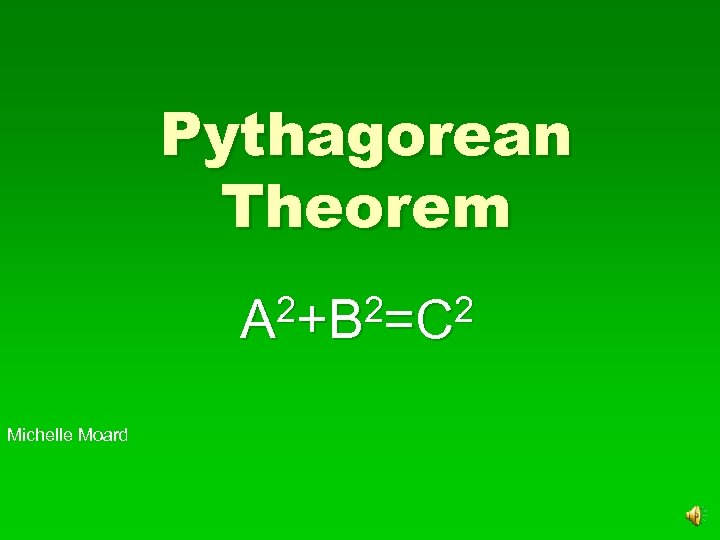Pythagorean Theorem 2+B 2=C 2 A Michelle Moard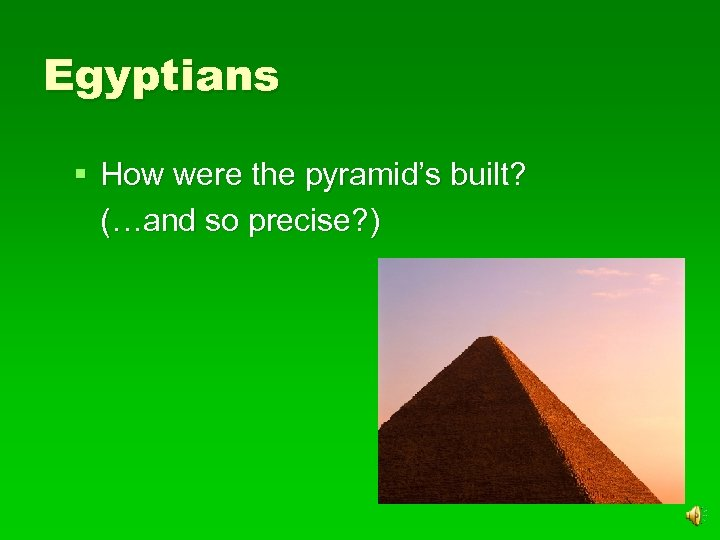Egyptians § How were the pyramid’s built? (…and so precise? )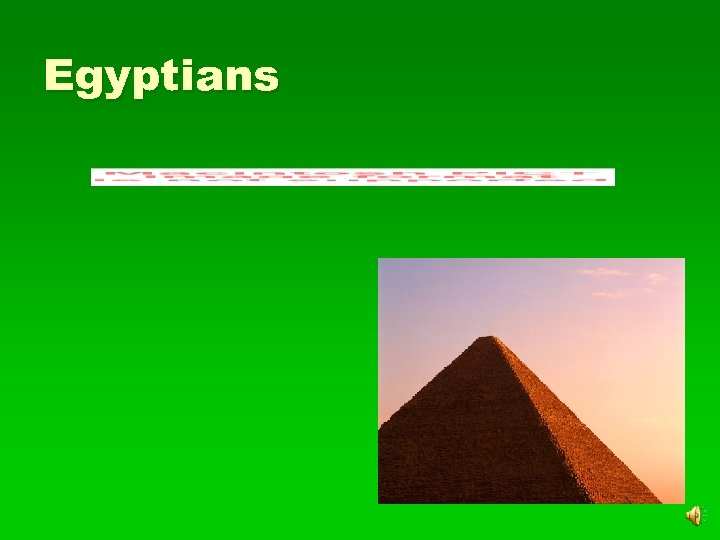Egyptians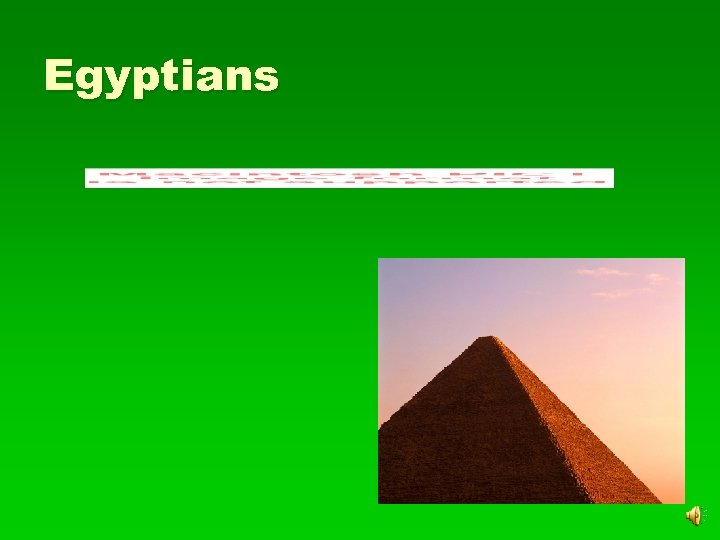Egyptians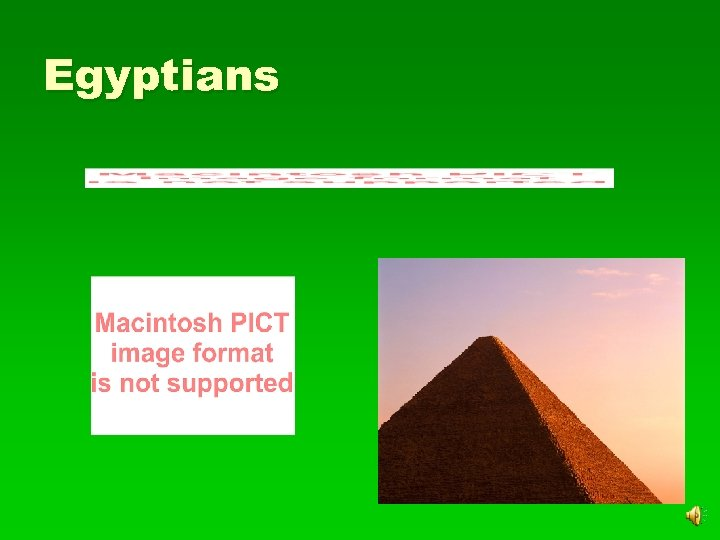Egyptians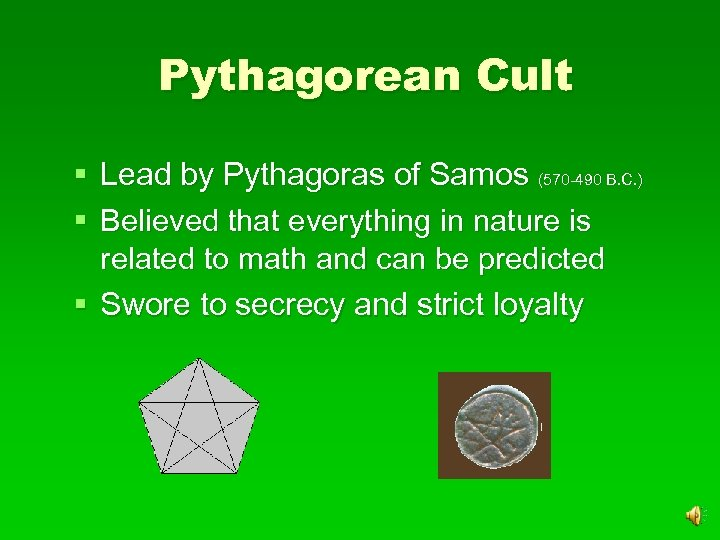Pythagorean Cult § Lead by Pythagoras of Samos (570 -490 B. C. ) § Believed that everything in nature is related to math and can be predicted § Swore to secrecy and strict loyalty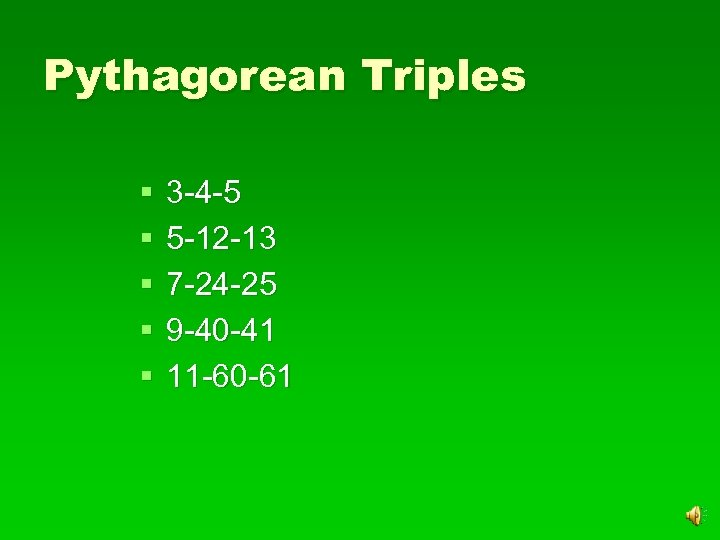Pythagorean Triples § § § 3 -4 -5 5 -12 -13 7 -24 -25 9 -40 -41 11 -60 -61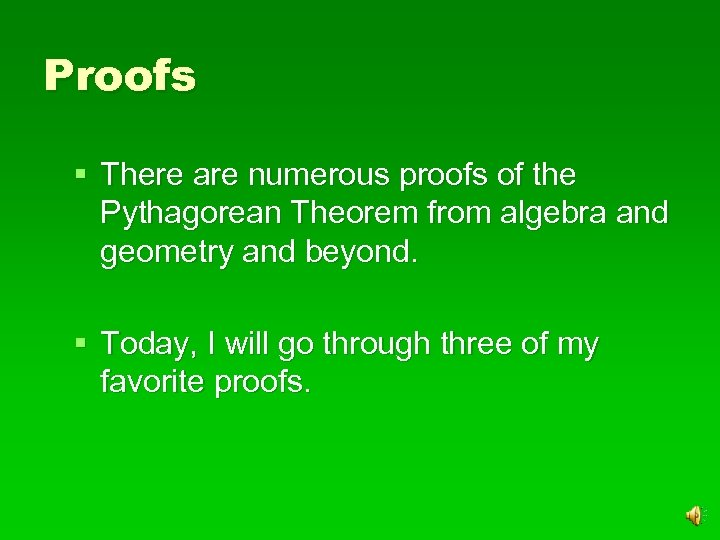Proofs § There are numerous proofs of the Pythagorean Theorem from algebra and geometry and beyond. § Today, I will go through three of my favorite proofs.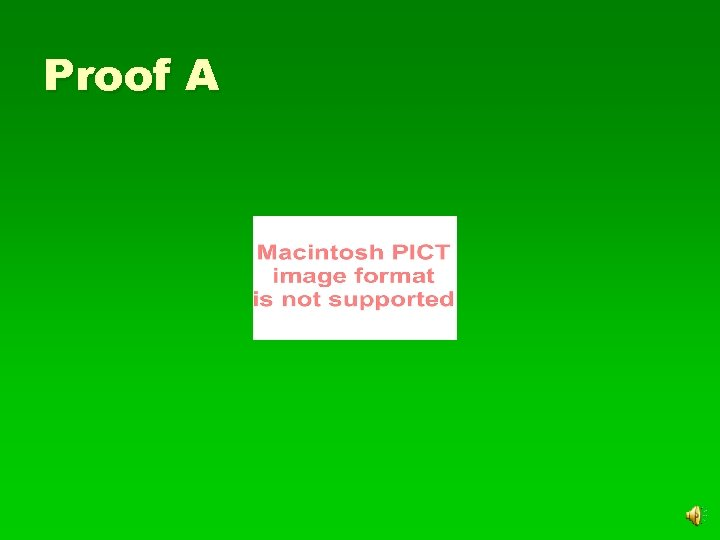Proof AProof AProof AProof AProof AProof AProof AProof AProof AProof AProof AProof AProof AProof AProof AProof AProof A A 2+B 2=C 2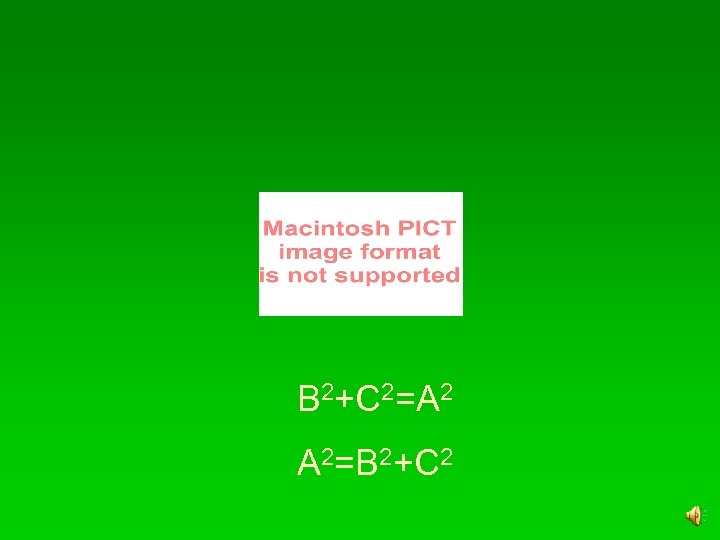B 2+C 2=A 2 A 2=B 2+C 2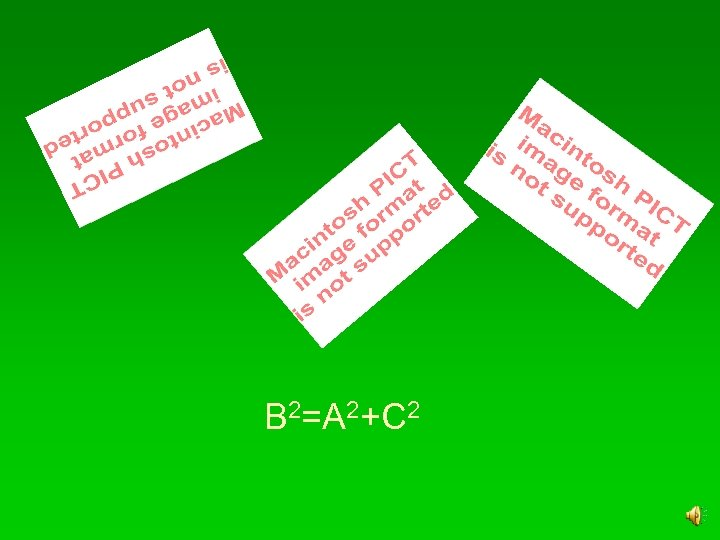B 2=A 2+C 2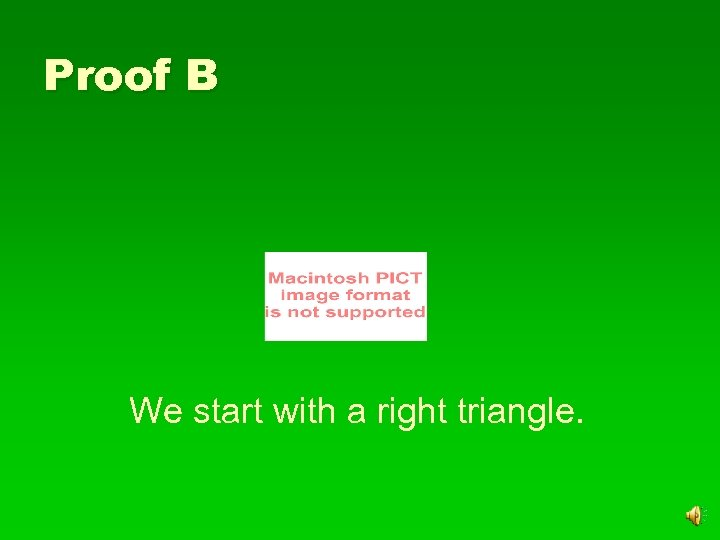Proof B We start with a right triangle.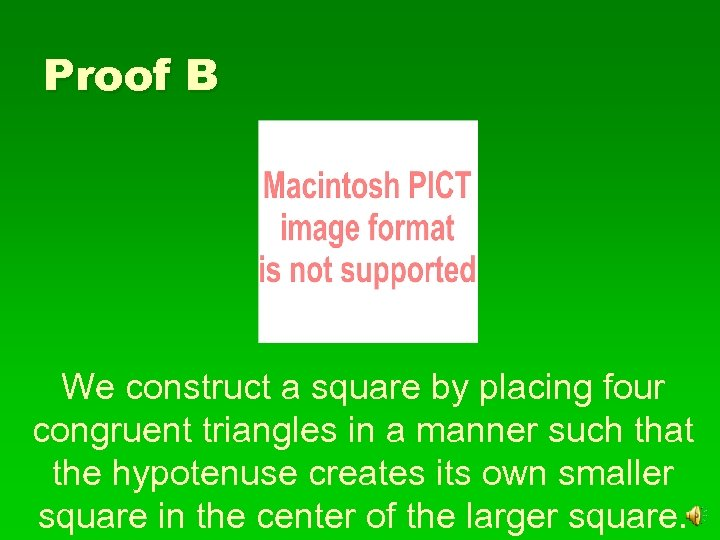Proof B We construct a square by placing four congruent triangles in a manner such that the hypotenuse creates its own smaller square in the center of the larger square.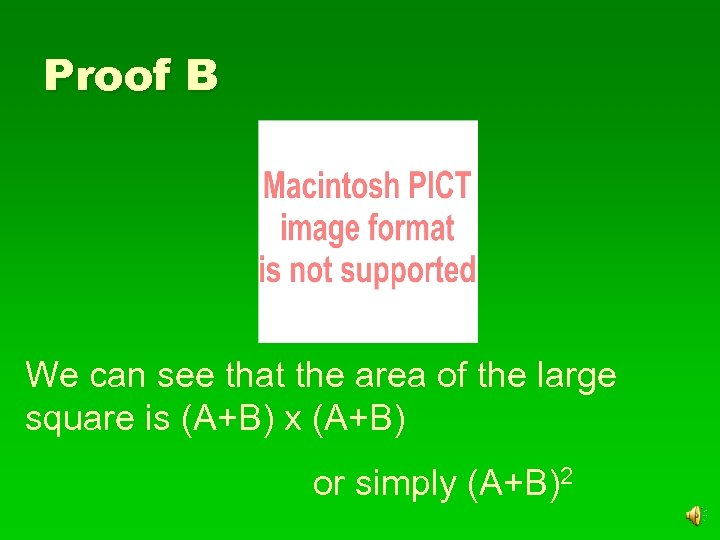Proof B We can see that the area of the large square is (A+B) x (A+B) or simply (A+B)2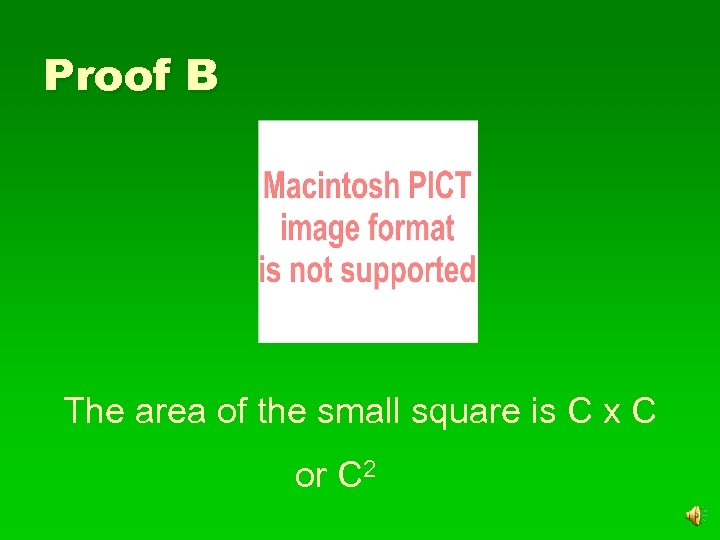Proof B The area of the small square is C x C or C 2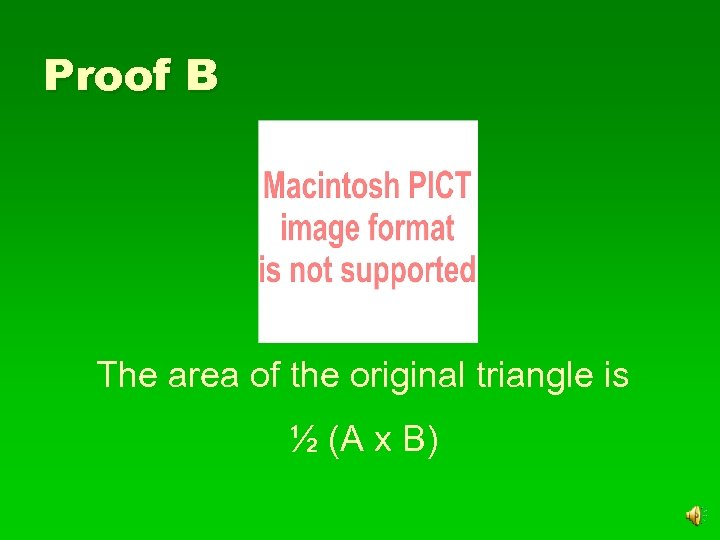Proof B The area of the original triangle is ½ (A x B)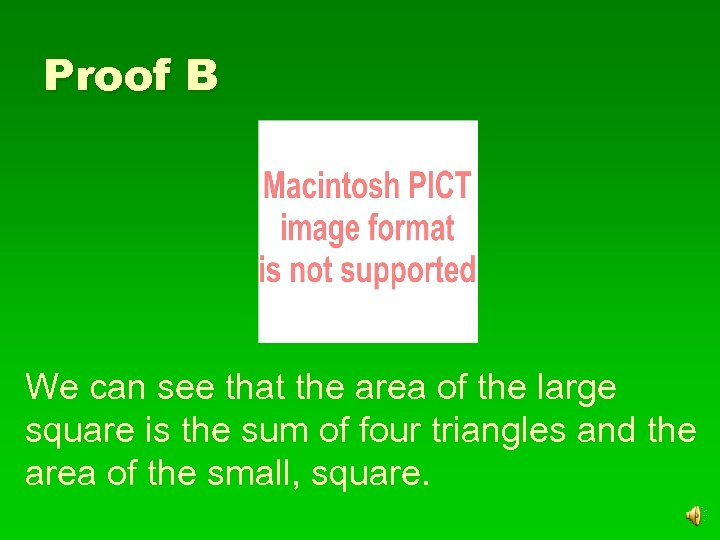Proof B We can see that the area of the large square is the sum of four triangles and the area of the small, square.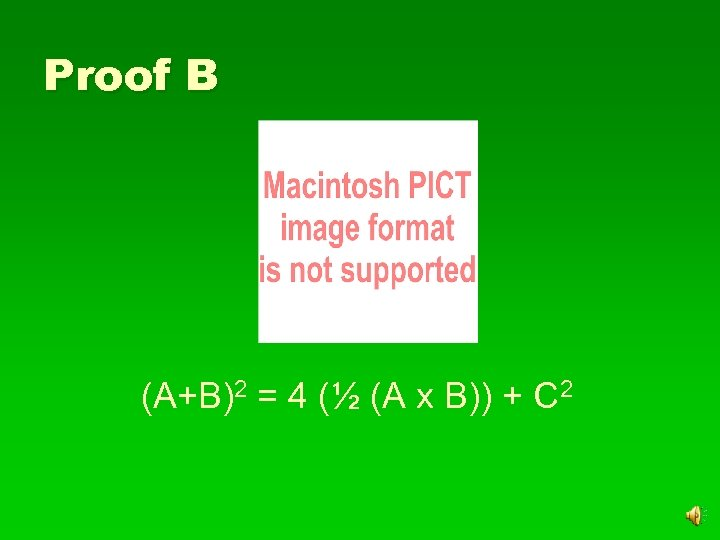Proof B (A+B)2 = 4 (½ (A x B)) + C 2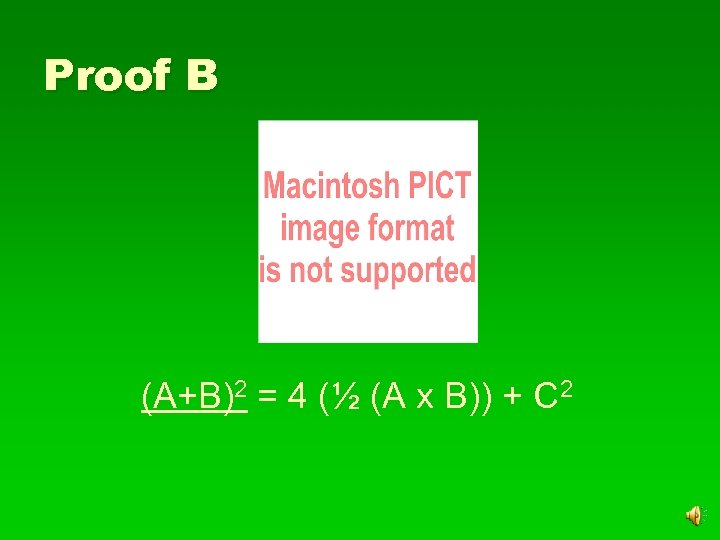Proof B (A+B)2 = 4 (½ (A x B)) + C 2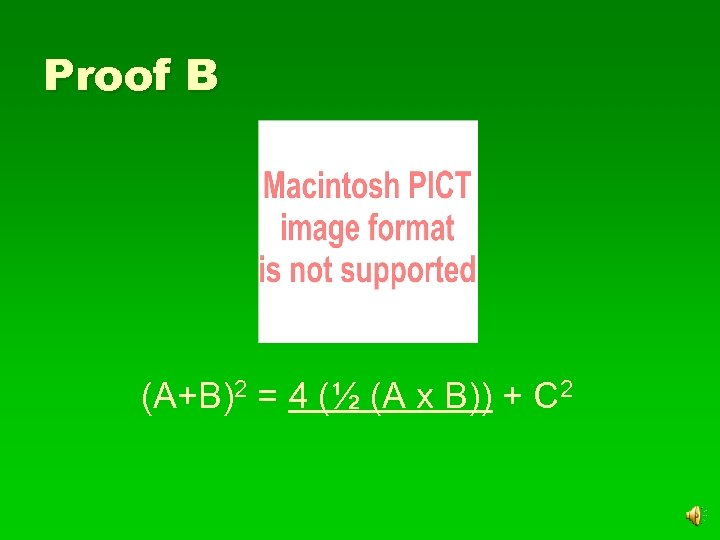Proof B (A+B)2 = 4 (½ (A x B)) + C 2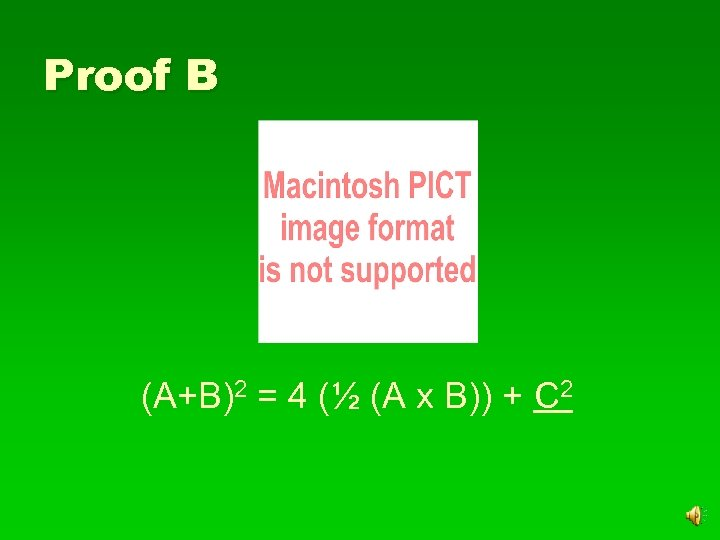Proof B (A+B)2 = 4 (½ (A x B)) + C 2Proof B (A+B)2 = 4 (½ (A x B)) + C 2Proof B (A+B)2 = 4 (½ (A x B)) + C 2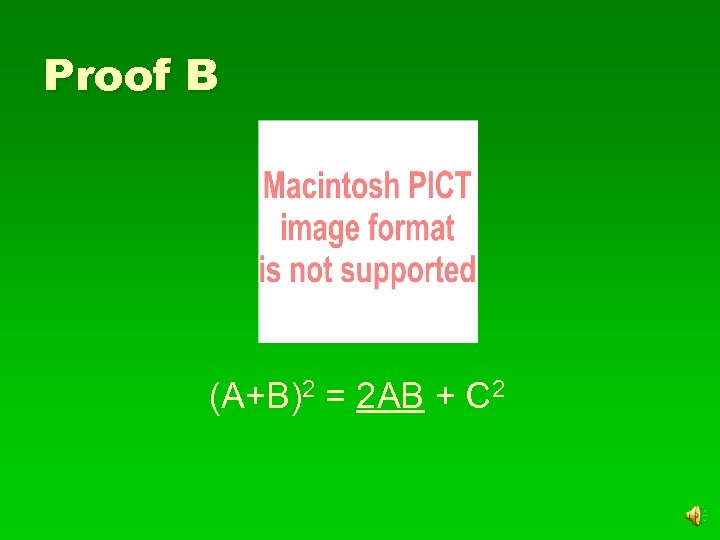Proof B (A+B)2 = 2 AB + C 2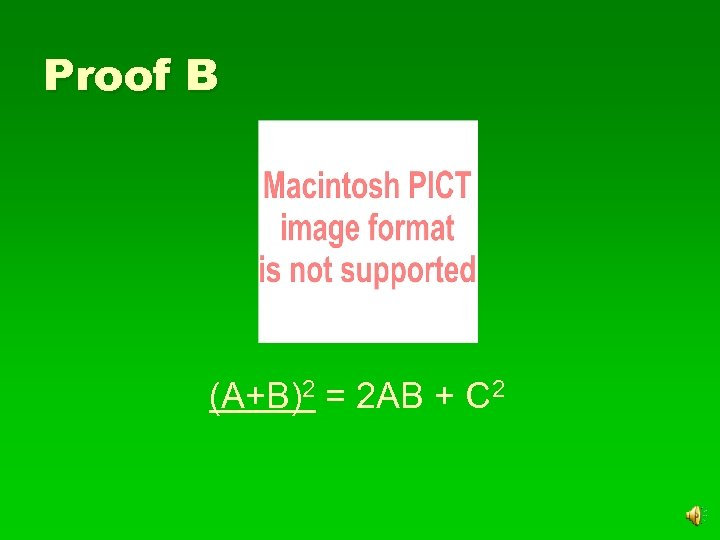Proof B (A+B)2 = 2 AB + C 2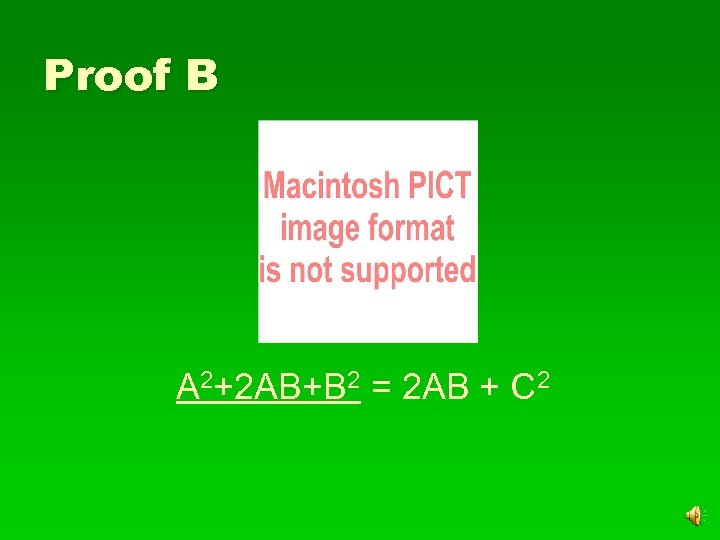Proof B A 2+2 AB+B 2 = 2 AB + C 2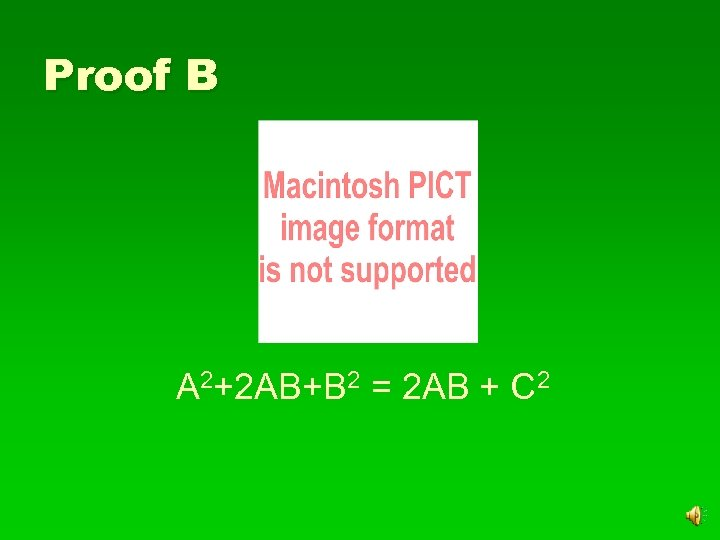Proof B A 2+2 AB+B 2 = 2 AB + C 2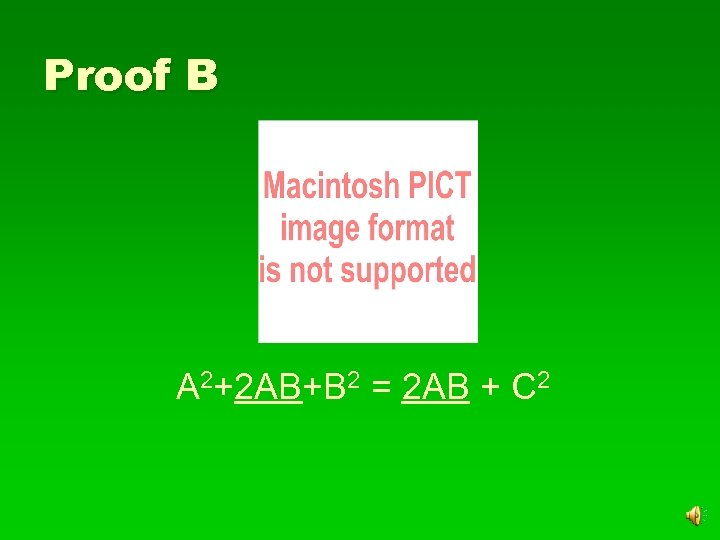Proof B A 2+2 AB+B 2 = 2 AB + C 2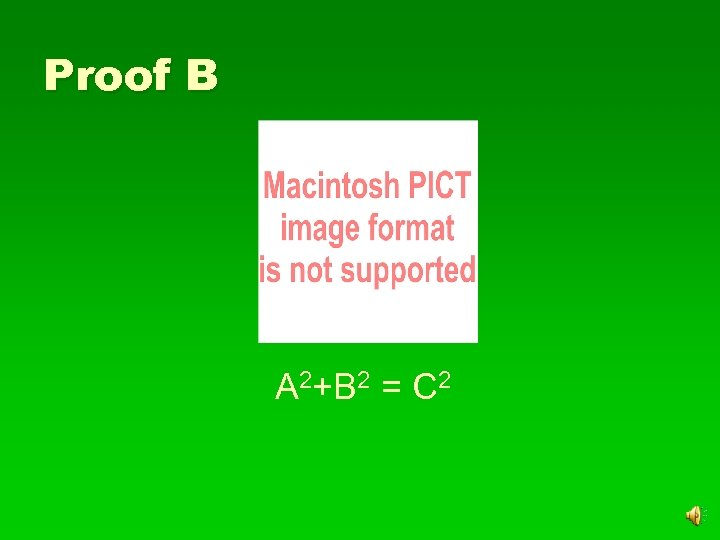Proof B A 2+B 2 = C 2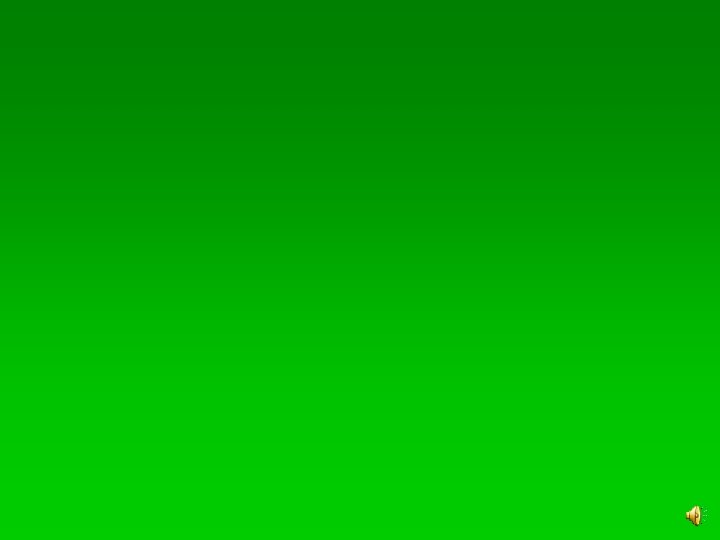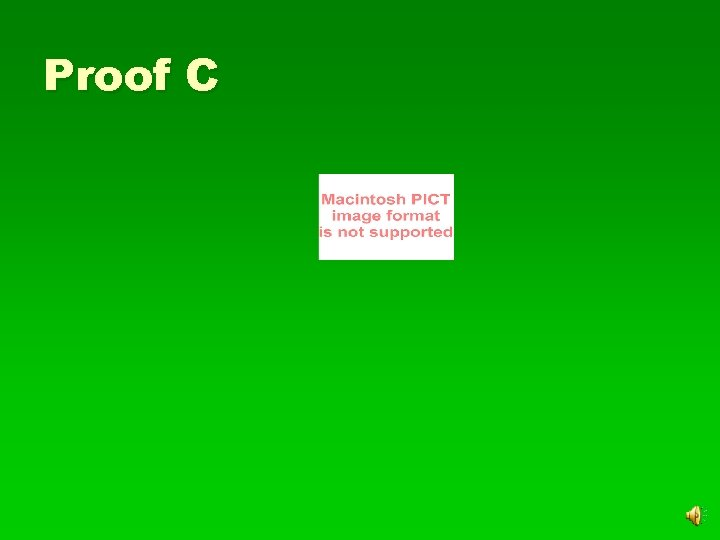Proof C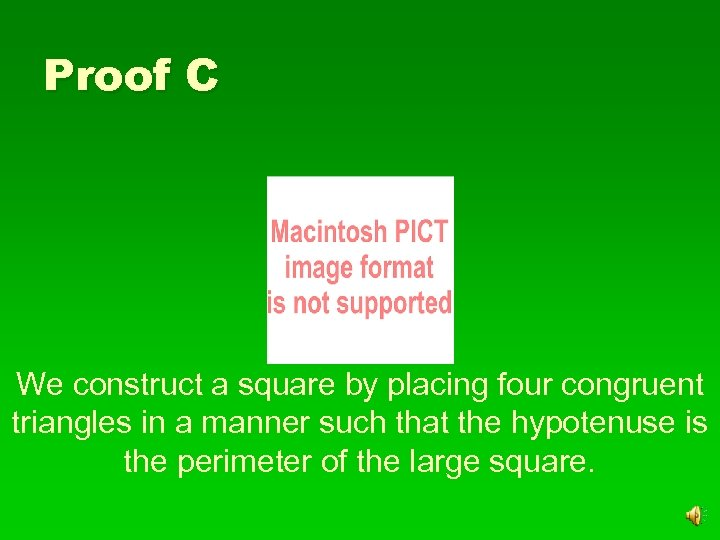Proof C We construct a square by placing four congruent triangles in a manner such that the hypotenuse is the perimeter of the large square.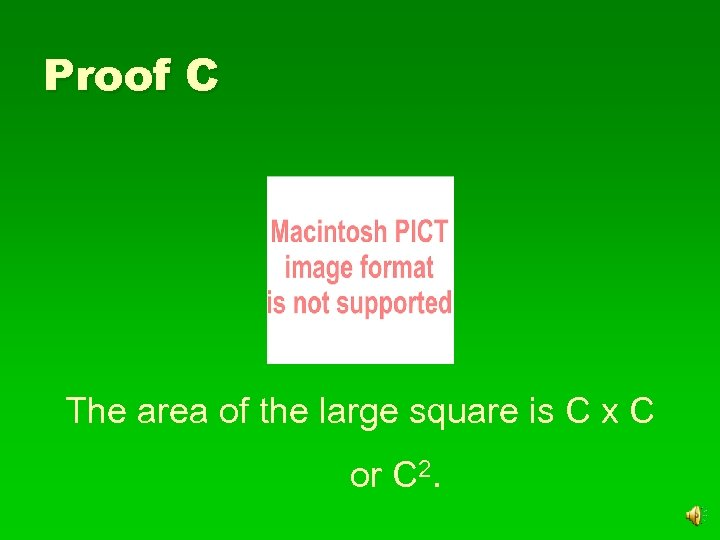Proof C The area of the large square is C x C or C 2.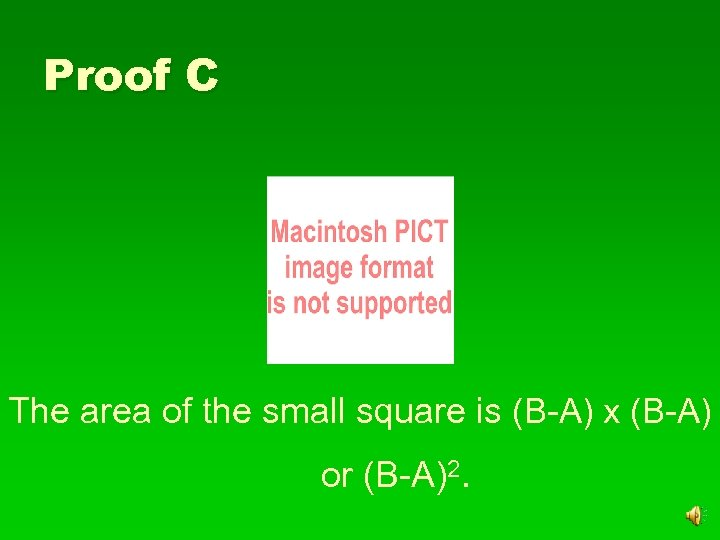Proof C The area of the small square is (B-A) x (B-A) or (B-A)2.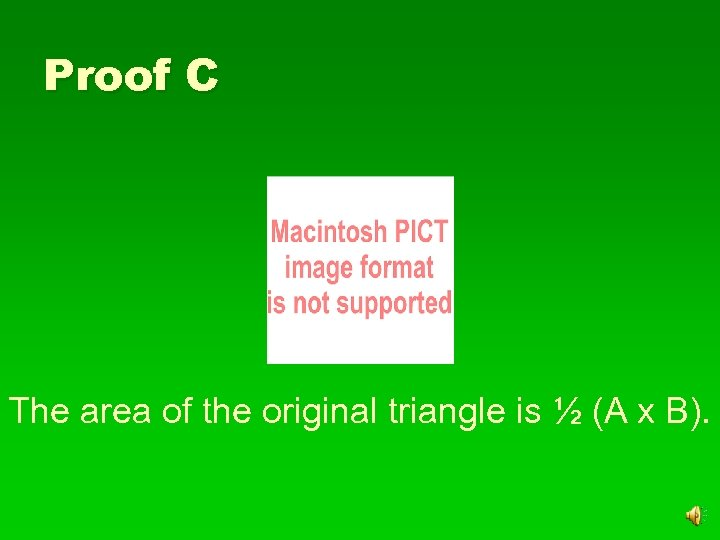Proof C The area of the original triangle is ½ (A x B).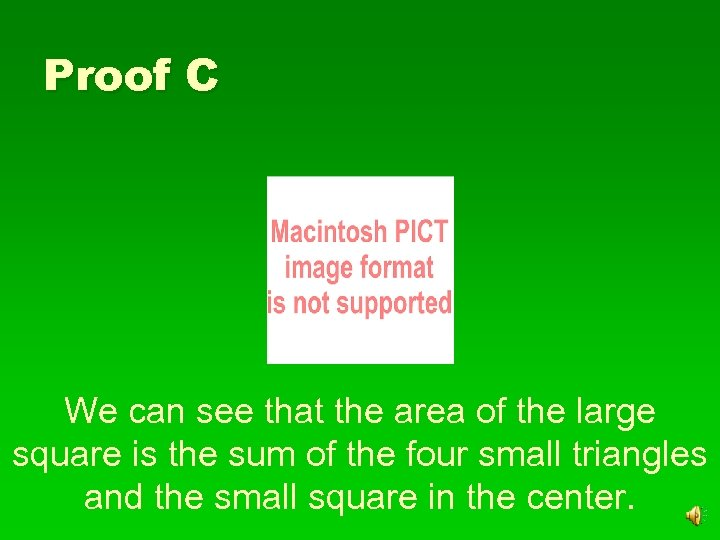Proof C We can see that the area of the large square is the sum of the four small triangles and the small square in the center.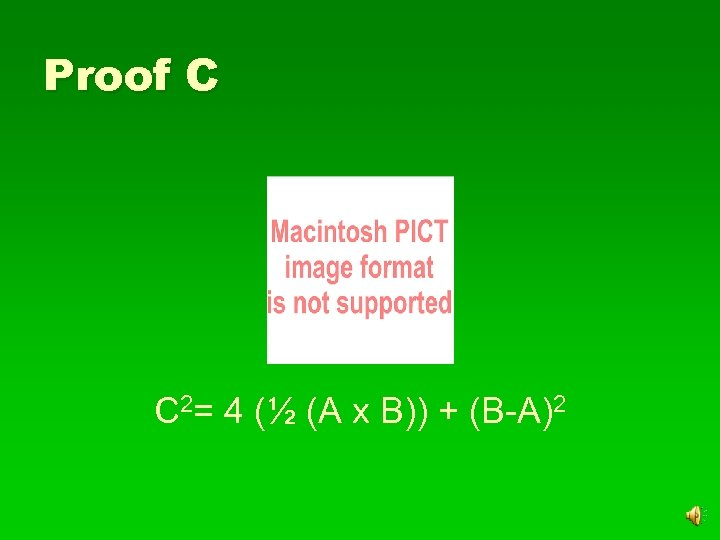Proof C C 2= 4 (½ (A x B)) + (B-A)2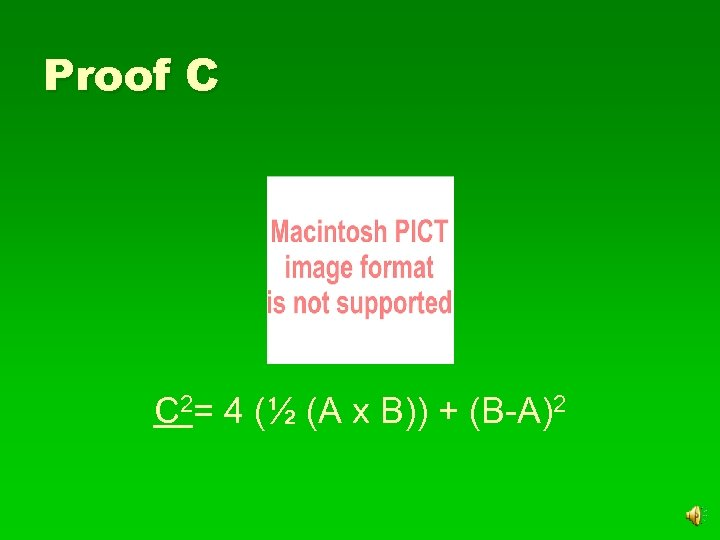Proof C C 2= 4 (½ (A x B)) + (B-A)2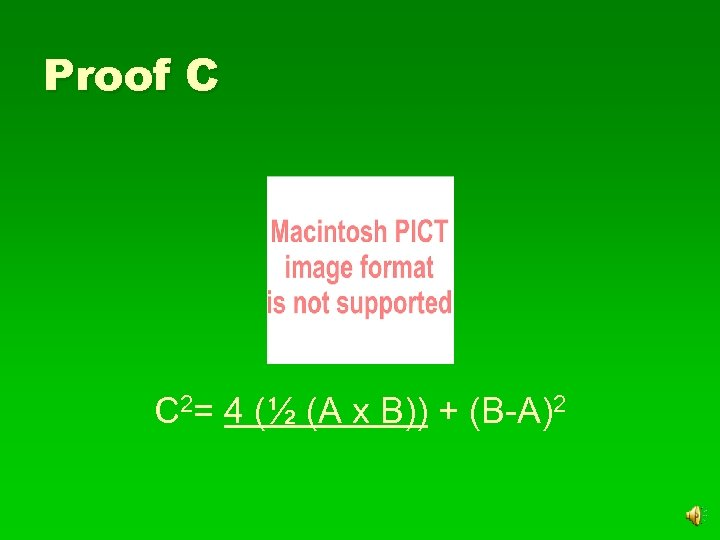Proof C C 2= 4 (½ (A x B)) + (B-A)2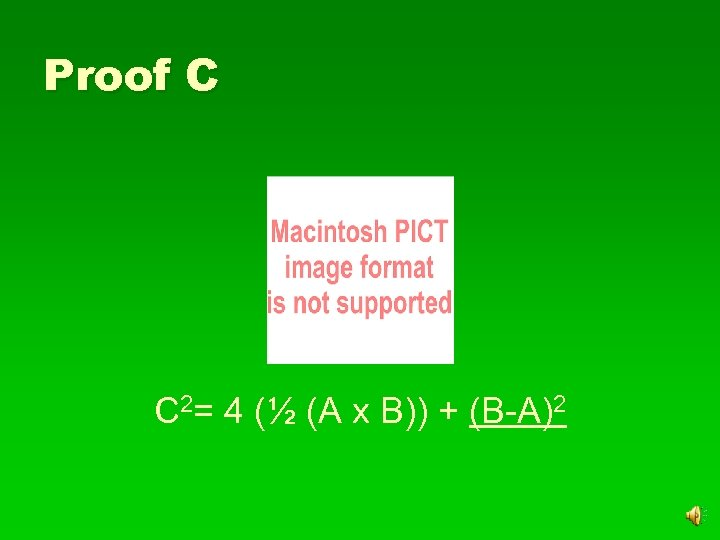Proof C C 2= 4 (½ (A x B)) + (B-A)2Proof C C 2= 4 (½ (A x B)) + (B-A)2Proof C C 2= 4 (½ (A x B)) + (B-A)2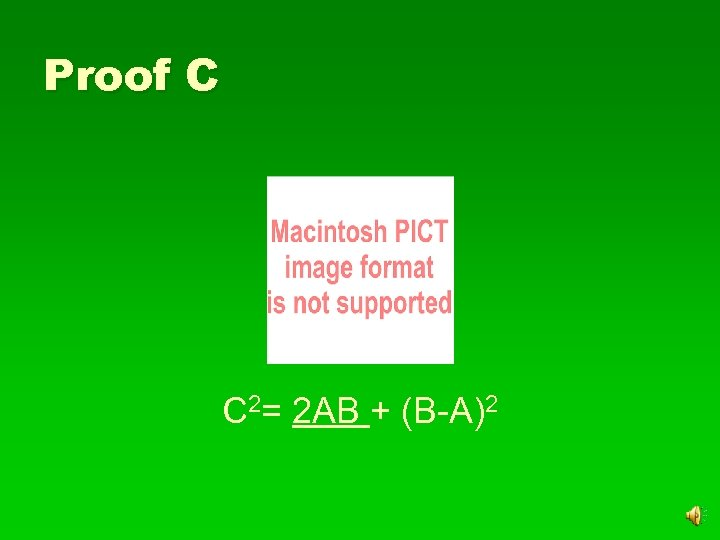Proof C C 2= 2 AB + (B-A)2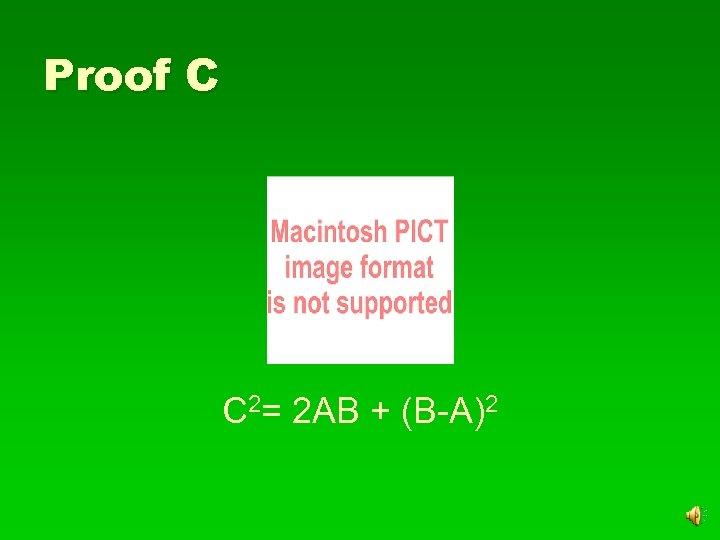Proof C C 2= 2 AB + (B-A)2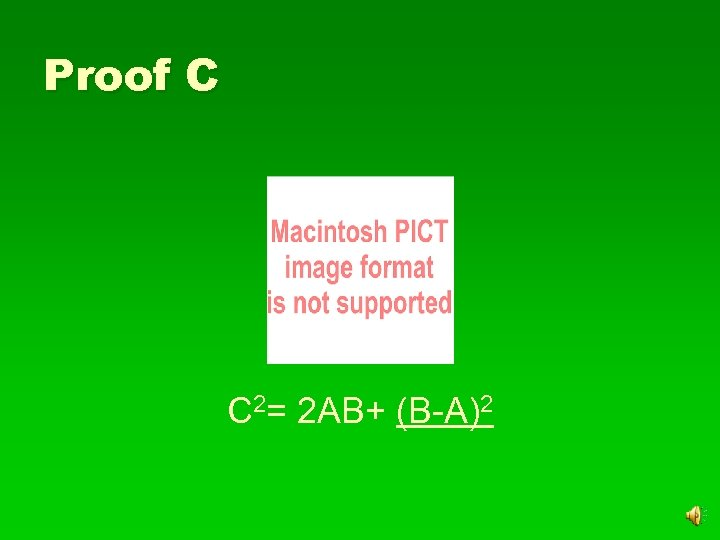Proof C C 2= 2 AB+ (B-A)2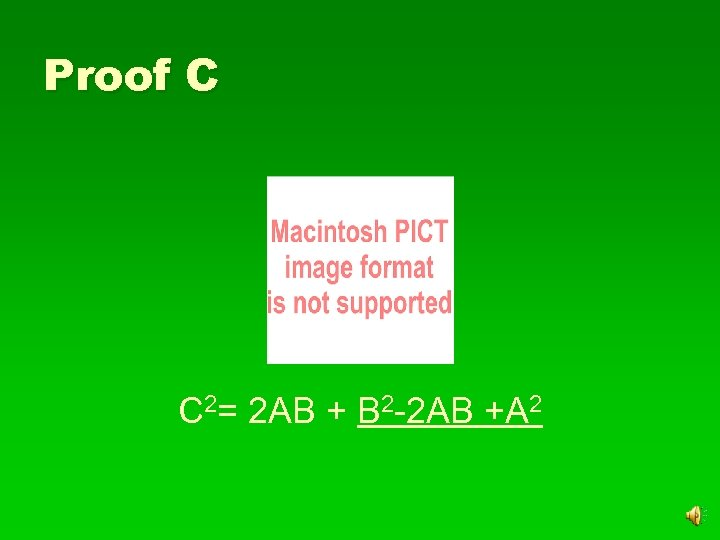Proof C C 2= 2 AB + B 2 -2 AB +A 2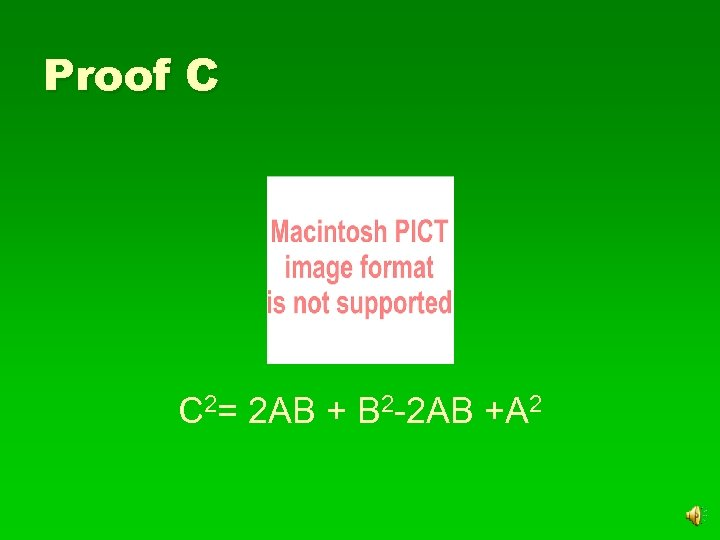Proof C C 2= 2 AB + B 2 -2 AB +A 2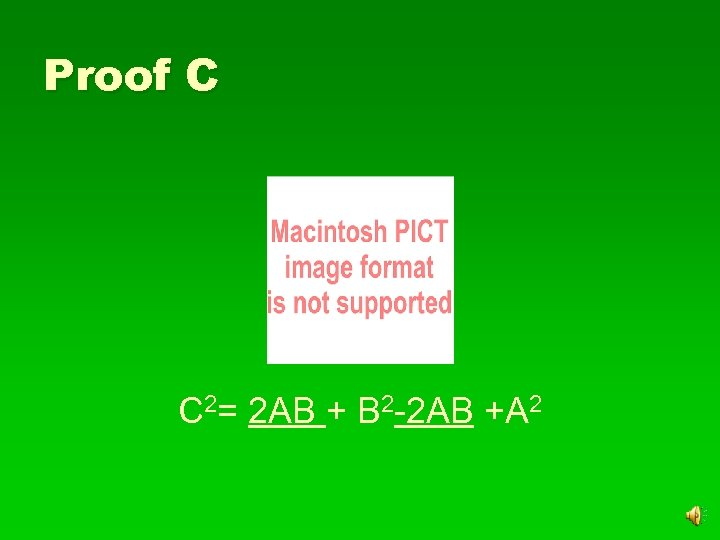Proof C C 2= 2 AB + B 2 -2 AB +A 2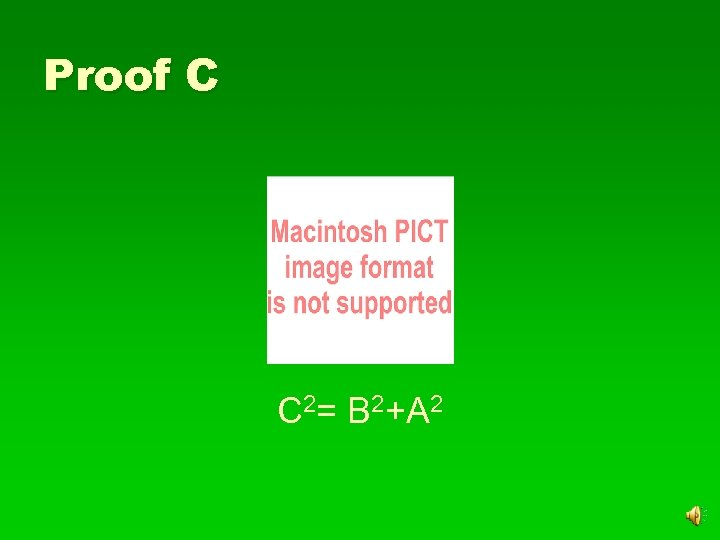Proof C C 2= B 2+A 2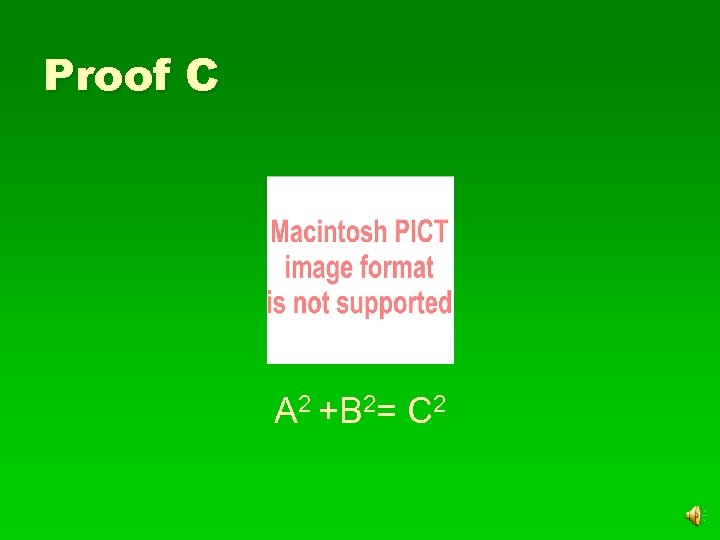Proof C A 2 +B 2= C 2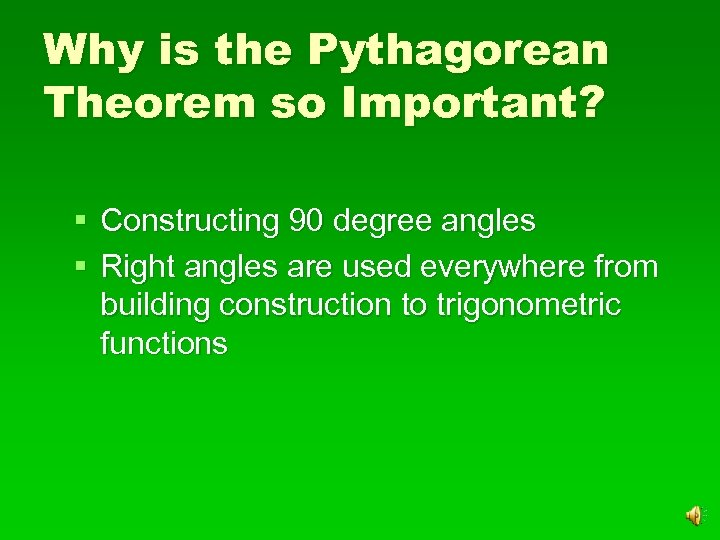Why is the Pythagorean Theorem so Important? § Constructing 90 degree angles § Right angles are used everywhere from building construction to trigonometric functions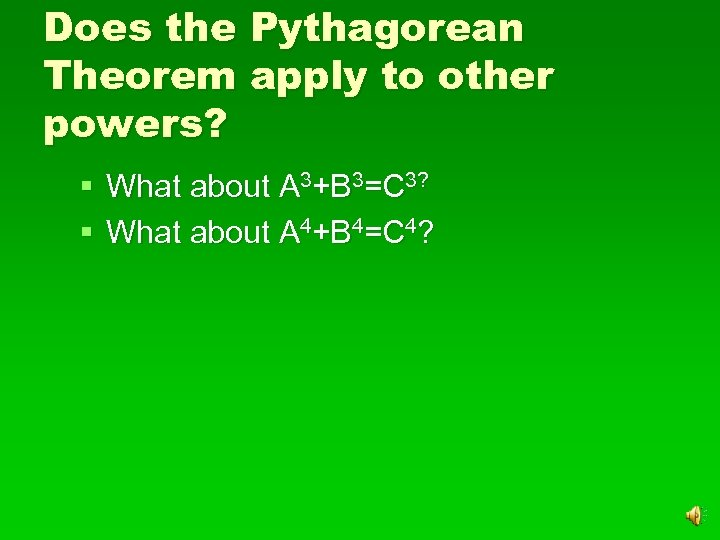Does the Pythagorean Theorem apply to other powers? § What about A 3+B 3=C 3? § What about A 4+B 4=C 4?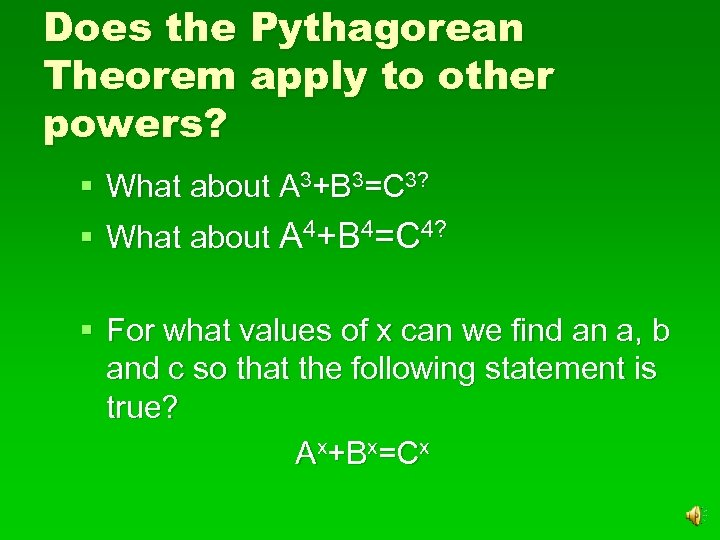Does the Pythagorean Theorem apply to other powers? § What about A 3+B 3=C 3? § What about A 4+B 4=C 4? § For what values of x can we find an a, b and c so that the following statement is true? Ax+Bx=Cx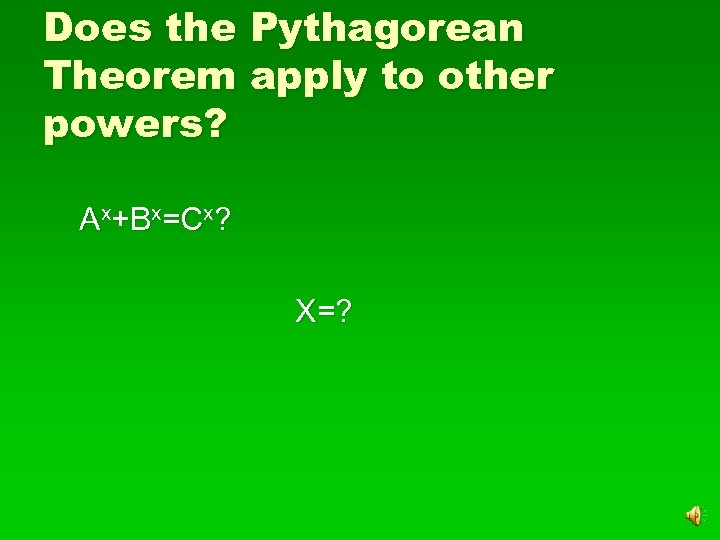Does the Pythagorean Theorem apply to other powers? Ax+Bx=Cx? X=?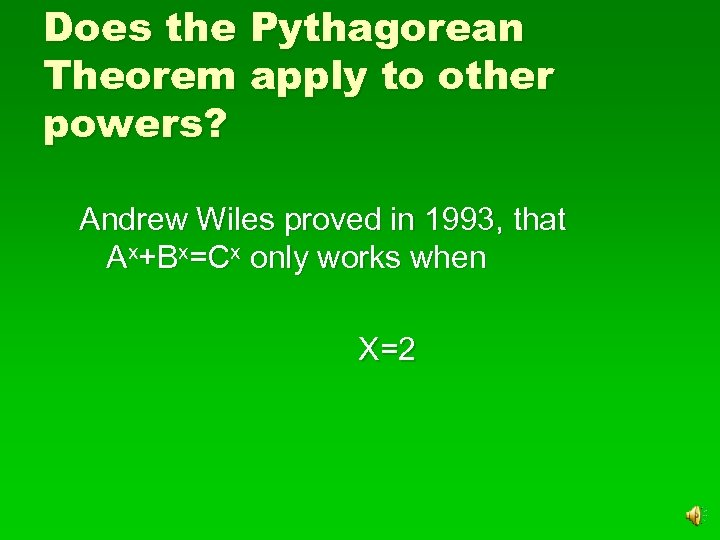Does the Pythagorean Theorem apply to other powers? Andrew Wiles proved in 1993, that Ax+Bx=Cx only works when X=2Pythagorean Theorem 2+B 2=C 2 A Michelle Moard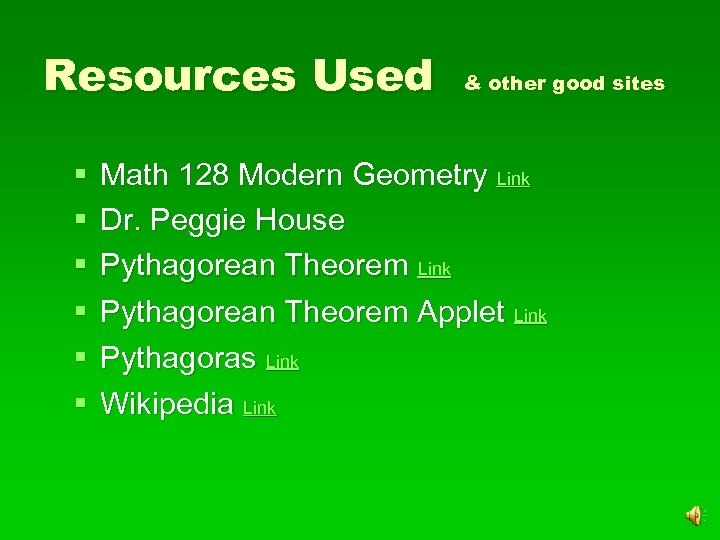Resources Used § § § & other good sites Math 128 Modern Geometry Link Dr. Peggie House Pythagorean Theorem Link Pythagorean Theorem Applet Link Pythagoras Link Wikipedia Link